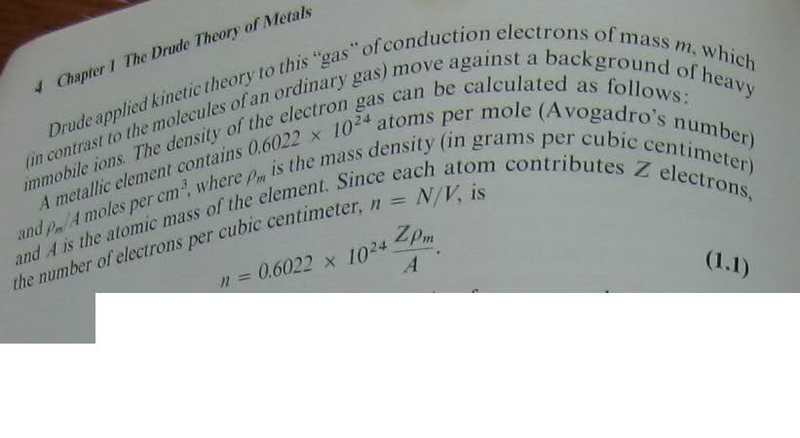# Semiconductors theory understanding nightmare1

nhrock3if we have a molecule or atom
then if we take avogadros number amount of this unit
then the amount will weight the atomic mass in grams.

$$\rho _m/A$$

rho is the density
A is the atomic mass

why their dinviding gives us moles per cube cantimeter
??

Last edited:

Okefenokee
In general, looking carefully at the units tends to help me understand physics. (BTW, solid state physics was the hardest subject for me.)

Anyway, we have this:

$$n = 0.6022 \times 10^{24} \frac{Z \rho_m}{A}$$

Physicists have a nasty habit of leaving out units when they write equations in text books. That didn't stop the physicists in my EE department from marking my grade down when I forgot to put units on my test answers. YOU'LL GET YOURS ONE DAY PHYSICISTS!!!

$$n (#electrons) = 0.6022 \times 10^{24} \frac{#atoms}{mole} \frac{Z \frac{#electrons}{atoms}\rho_m \frac{mass}{cm^3}}{A \frac{mass}{mole}}$$

You can see how the units cancel now. Does that help?

nhrock3
thanks :)

this is only the first question among many
to understand 4 chapters
thanks again :)

nhrock3
what is N?
in the N/V formula

Okefenokee
Man, I can do anything these days without making an error. The correct units were:

$$n \frac{electrons}{cm^3}= 0.6022 \times 10^{24} \frac{#atoms}{mole} \frac{Z \frac{#electrons}{atoms}\rho_m \frac{mass}{cm^3}}{A \frac{mass}{mole}}$$

$$n = \frac{N(electrons)}{V(cm^3)}$$